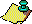Contents Next: Existence of stopping Up: Generalizations of problem. Previous: Generalizations of problem.

J. H. Conway  proved the remarkable result that a simple generalization of the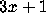problem is algorithmically undecidable. He considers the class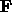of periodically piecewise linear functions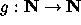having the structure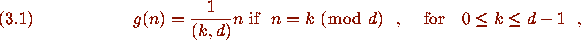specified by the nonnegative integers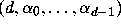. These are exactly the functionssuch that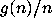is periodic.

### Theorem O

(Conway). For every partial recursive function f defined on a subset D of the natural numbers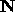there exists a functionsuch that

(1)is periodic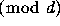for some d and takes rational values.

(2) There is some iterate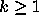such that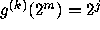for some j if and only if m is in D.

(3)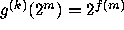for the minimalsuch that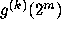is a power of 2.

Conway's proof actually gives in principle a procedure for explicitly constructing such a function g given a description of a Turing machinethat computes f. He carried out this procedure to find a function g associated to a particular partial recursive function f having the property that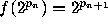, where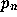is the nth prime; this is described in Guy . By choosing a particular partial recursive function whose domain is not a recursive subset of, e.g., a function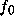that encodes the halting problem for Turing machines, we obtain the following corollary of Theorem O.

### Theorem P

(Conway). There exists a particular, explicitly constructible function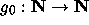such that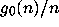is periodicfor a finite modulus d and takes rational values, for which there is no Turing machine that, when given n, always decides in a finite number of steps whether or not some iterate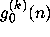withis a power of 2.Contents Next: Existence of stopping Up: Generalizations of problem. Previous: Generalizations of problem.